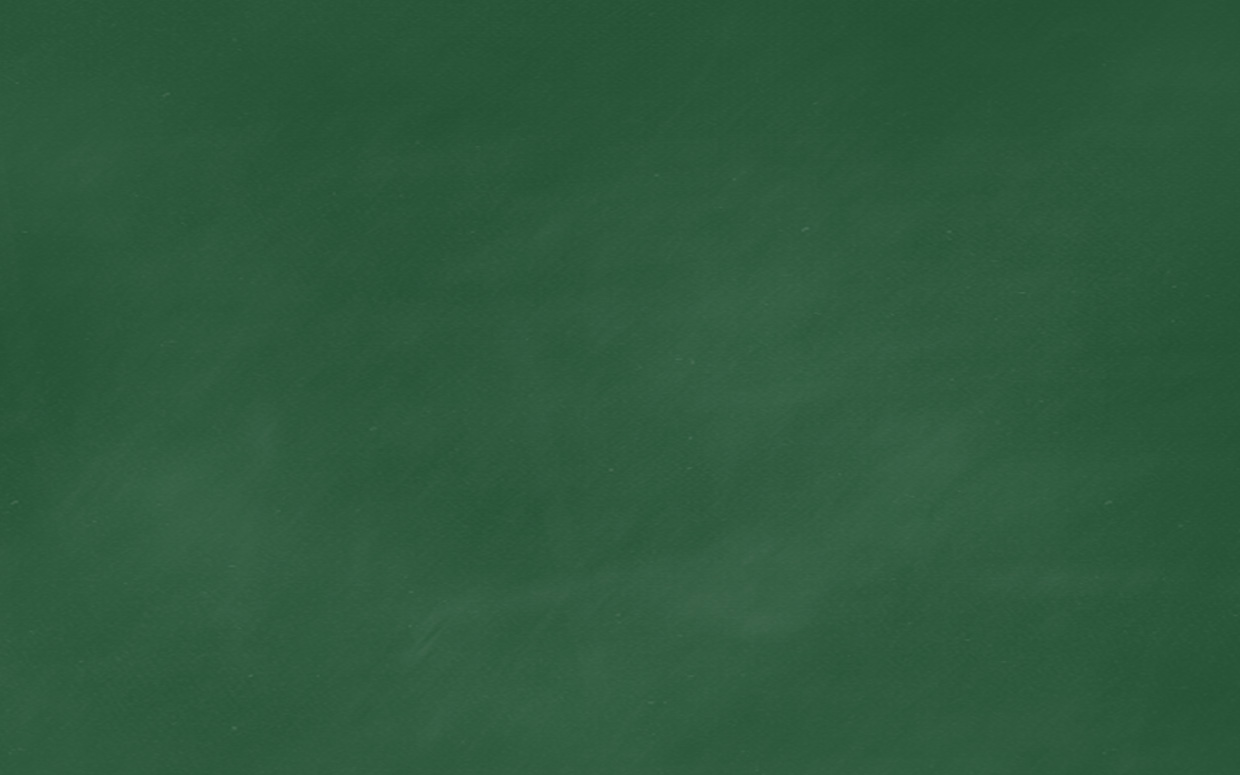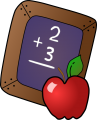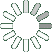Puzzle Command
Daily Puzzle
Alternate Reality Math
April 1, 2015In an alternate reality Math is still base 10 but the digits that are used represent different digits than you are used to.

1 is 9, 2 is 8, 3 is 4, 4 is 5, 5 is 3, 6 is 1, 7 is 2, 8 is 7, 9 is 0, and 0 is 6.

For example:

8 + 9 = 8 [7 + 0 = 7] 10 + 10 = 908 [96 + 96 = 192]

Use the different digit representations to calculate the following fact:

(3 * 89) + (55 - 66) = ?

Puzzle Notes
1 is 9, 2 is 8, 3 is 4, 4 is 5, 5 is 3, 6 is 1, 7 is 2, 8 is 7, 9 is 0, and 0 is 6.
Answer must be in the same key as the alternate reality
Enter in your solution below and click submit.